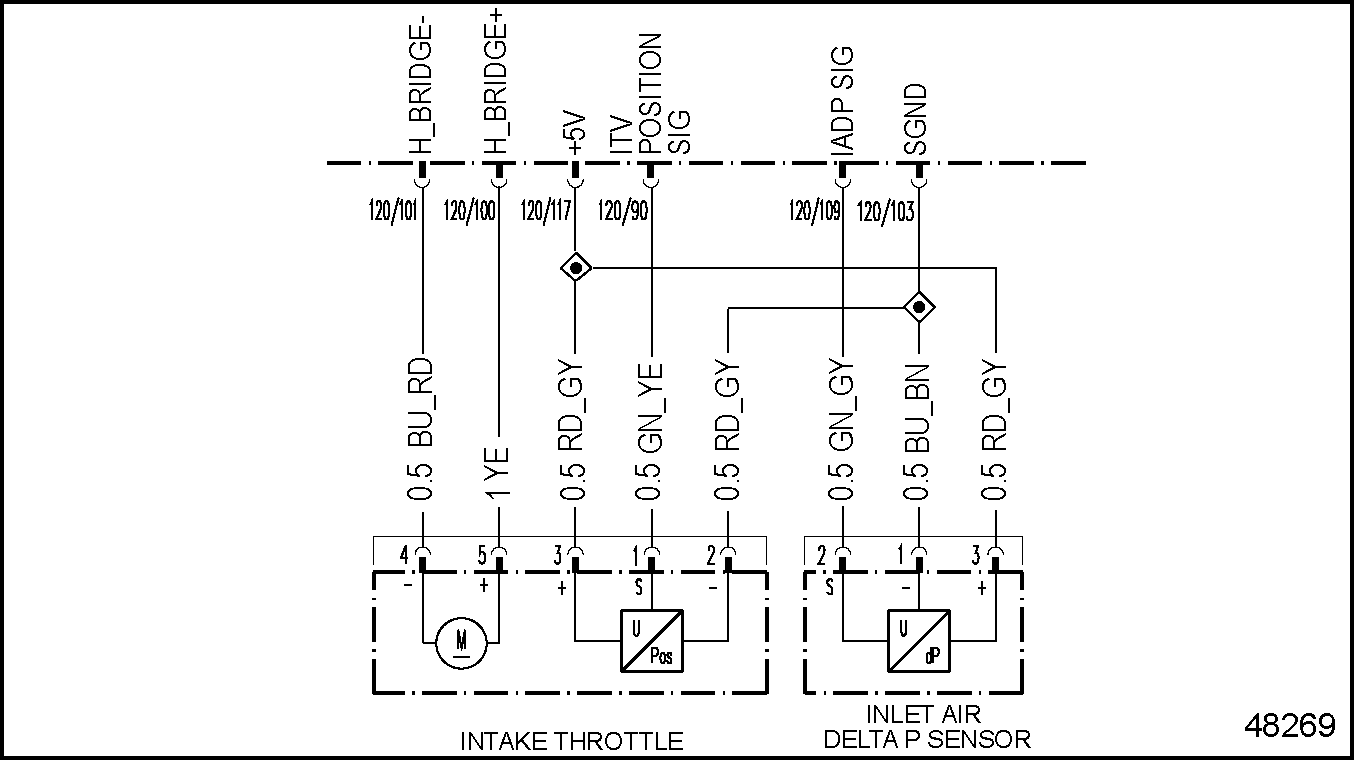MBE 900 DDEC VI Troubleshooting

# Section 131.3 SPN 4226/FMI 3

### Section 131.3 SPN 4226/FMI 3

This diagnosis is typically an open circuit.‪

#### Section 131.3.1 Open Circuit Check

Check as follows:‪

1. Check for multiple codes.
1. If 51/3 and 4226/3 are present, repair the open between pin 103 of the 120–pin MCM connector and pin 2 of the Inlet Air Delta P Sensor and the Intake Throttle Valve. See Figure "Inlet Air Delta P Sensor" . Refer to "131.3.1.1 Verify Repairs" .Figure 1. Inlet Air Delta P Sensor

2. If only 4226/3 is present, go to step 2 .
2. Disconnect the Inlet Air Delta P Sensor.
3. Measure the resistance across pins 1 and 2 of the Inlet Air Delta P Sensor.
1. If the resistance is greater than 200k Ω, replace the Inlet Air Delta P Sensor.
2. If the resistance is less than 200k Ω, go to step 4 .
4. Measure the resistance across pins 1 and 3 of the Inlet Air Delta P Sensor.
1. If the resistance is greater than 50k Ω, replace the Inlet Air Delta P Sensor.
2. If the resistance is less than 50k Ω, go to step 5 .
5. Turn the ignition ON (key ON, engine OFF).
6. Measure the voltage between pins 1 and 2 of the Inlet Air Delta P Sensor harness connector.
1. If the voltage is between 4.5 and 5.5 volts, go to step 8 .
2. If the voltage is less than 4.5 volts, go to step 7 .
7. Measure the voltage between pin 1 of the Inlet Air Delta P Sensor harness connector and ground.
1. If the voltage is between 4.5 and 5.5 volts, repair the open circuit between pin 2 of the Inlet Air Delta P Sensor harness connector and pin 103 of the 120–pin MCM connector. See Figure "120–pin MCM Connector" . Refer to "131.3.1.1 Verify Repairs" .
2.Figure 2. 120–pin MCM Connector

3. If the voltage is less than 4.5 volts, repair the open circuit between pin 1 of the Inlet Air Delta P Sensor harness connector and pin 117 of the 120–pin MCM connector. Refer to "131.3.1.1 Verify Repairs" .
8. Turn the ignition OFF.
9. Disconnect the 120–pin MCM connector.
10. Measure the resistance between pins 1 and 3 of the Inlet Air Delta P Sensor harness connector.
1. If the resistance is greater than 5 Ω, go to step 11 .
2. If the resistance is less than 5 Ω, repair the short in wires between pins 1 and 3 of the Inlet Air Delta P Sensor harness connector and pin 109 of the 120–pin MCM connector. Refer to "131.3.1.1 Verify Repairs" .
11. Measure the resistance between pin 3 of the Inlet Air Delta P Sensor harness connector and pin 109 of the 120–pin MCM connector.
1. If the resistance is greater than 3 Ω, repair the open between pin 3 of the Inlet Air Delta P Sensor harness connector and pin 109 of the 120–pin MCM connector. Refer to "131.3.1.1 Verify Repairs" .
2. If the resistance is less than 3 Ω, repeat steps 2 through 11 . If the results are the same, contact the Detroit Diesel Customer Support Center (313–592–5800).
##### Section 131.3.1.1 Verify Repairs

Verify repairs as follows:‪

1. Turn ignition OFF.
2. Reconnect any electrical connections that were disconnected to perform the diagnosis.